NEET  >  Self-Inductance

# Self-Inductance - Notes | Study Physics Class 12 - NEET

 1 Crore+ students have signed up on EduRev. Have you?

6. Self induction

Self induction is induction of emf in a coil due to its own current change. Total flux N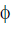passing through a coil due to its own current is proportional to the current and is given as N= L i where L is called coefficient of self induction or inductance. The inductance L is purely a geometrical property i.e., we can tell the inductance value even if a coil is not connected in a circuit. Inductance depends on the shape and size of the loop and the number of turns it has.

If current in the coil changes by ΔI in a time interval Δt, the average emf induced in the coil is given as

ε =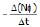=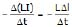The instantaneous emf is given as

ε = -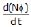= -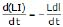S.I unit of inductance is wb/amp or Henry (H)

L - self inductance is +ve Quantity.

L depends on : (1) Geometry of loop

(2) Medium in which it is kept. L does not depend upon current. L is a scalar Quantity.

Brain Teaser

If a circuit has large self-inductance, what inference can you draw about the circuit.

6.1 Self Inductance of solenoid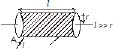Let the volume of the solenoid be V, the number of turns per unit length be n. Let a current I be flowing in the solenoid. Magnetic field in the solenoid is given as B = μ0 nl. The magnetic flux through one turn of solenoid f = μ0 n l A.

The total magnetic flux through the solenoid = N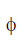= N μ0 n l A

= μ0 n2 l A l

Therefore, L = μ0 n2 l A = μ0 n2 V= μ0 n i pr2 (n l)

L =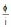= μ0 n2 πr2 l

Inductance per unit volume = μ0 n2

Ex.38 The current in a coil of self-inductance L = 2H is increasing according to the law i = 2 sin t2. Find the amount of energy spent during the period when the current changes from 0 to 2 ampere.

Sol. Let the current be 2 amp at t = τ

Then 2 = 2 sin τ2 ⇒ τ =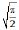When the instantaneous current is i, the self induced emf is L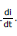. If the amount of charge that is displaced in time dt is dθ, then the elementary work done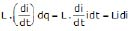W =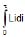=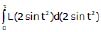W =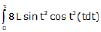=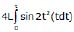Let θ = 2t2

Differentiating dθ = 4t dt

Therefore, W =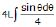= L (- cosθ) = - L cos 2t2

W =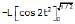= 2 L = 2 × 2 = 4 joule

7. Inductor

It is represent by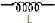electrical equivalence of loop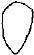⇒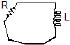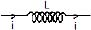If current i through the inductor is increasing the induced emf will oppose the increase in current and hence will be opposite to the current. If current i through the inductor is decreasing the induced emf will oppose the decrease in current and hence will be in the direction of the current.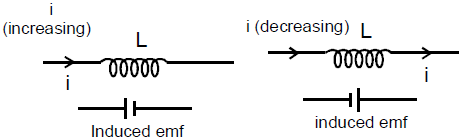Over all result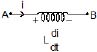VA - L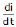= VB

Note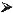If there is a resistance in the inductor (resistance of the coil of inductor) then :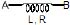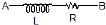Ex.39 A B is a part of circuit. Find the potential difference vA - vB if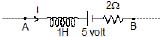(i) current i = 2A and is constant

(ii) current i = 2A and is increasing at the rate of 1 amp/sec.

(iii) current i = 2A and is decreasing at the rate 1 amp/sec.

Sol.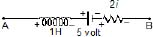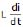=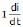writing KVL from A to B

VA - 1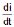- 5 - 2 i = VB

(i) Put i = 2,= 0

VA - 5 - 4 = VB    Therefore, VA - VB = 9 volt

(ii) Put i = 2,= 1 ;

VA - 1 - 5 - 4 = VB or VA - VB = 10 V0

(iii) Put i = 2,= - 1

VA + 1 - 5 - 2 × 2 = VB

VA = 8 volt

Ex.40 Find current i, i1 and i2 in the following circuit.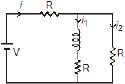Sol. at t = 0

ii2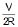and i1 = 0

at t = ∞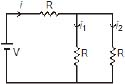⇒ i1 = i2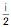=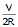7.1 Energy stored in an inductor :

If current in an inductor at an instant is i and is increasing at the rate di/dt, the induced emf will oppose the current. Its behaviour is shown in the figure.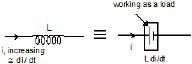Power consumed by the inductor = i LEnergy consumed in dt time = i Ldt

Therefore, total energy consumed as the current increases from 0 to I =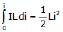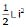⇒ U =Note :This energy is stored in the magnetic field with energy density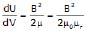Total energy U =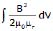Ex.41 Find out the energy per unit length ratio inside the solid long wire having current density J.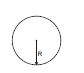Sol. Take a ring of radius r and thickness dr as an element inside the wire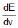=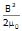using B =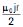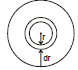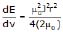⇒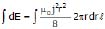⇒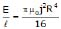The document Self-Inductance - Notes | Study Physics Class 12 - NEET is a part of the NEET Course Physics Class 12.
All you need of NEET at this link: NEET

## Physics Class 12

157 videos|452 docs|213 tests
 Use Code STAYHOME200 and get INR 200 additional OFF

## Physics Class 12

157 videos|452 docs|213 tests

### How to Prepare for NEET

Read our guide to prepare for NEET which is created by Toppers & the best Teachers

Track your progress, build streaks, highlight & save important lessons and more!

,

,

,

,

,

,

,

,

,

,

,

,

,

,

,

,

,

,

,

,

,

;